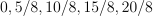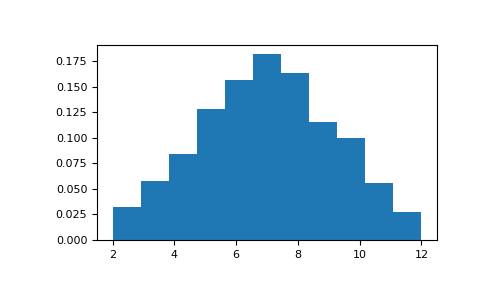/NumPy 1.14

# numpy.random.random_integers

`numpy.random.random_integers(low, high=None, size=None)`

Random integers of type np.int between `low` and `high`, inclusive.

Return random integers of type np.int from the “discrete uniform” distribution in the closed interval [`low`, `high`]. If `high` is None (the default), then results are from [1, `low`]. The np.int type translates to the C long type used by Python 2 for “short” integers and its precision is platform dependent.

This function has been deprecated. Use randint instead.

Deprecated since version 1.11.0.

Parameters: low : int Lowest (signed) integer to be drawn from the distribution (unless `high=None`, in which case this parameter is the highest such integer). high : int, optional If provided, the largest (signed) integer to be drawn from the distribution (see above for behavior if `high=None`). size : int or tuple of ints, optional Output shape. If the given shape is, e.g., `(m, n, k)`, then `m * n * k` samples are drawn. Default is None, in which case a single value is returned. out : int or ndarray of ints `size`-shaped array of random integers from the appropriate distribution, or a single such random int if `size` not provided.

`random.randint`
Similar to `random_integers`, only for the half-open interval [`low`, `high`), and 0 is the lowest value if `high` is omitted.

#### Notes

To sample from N evenly spaced floating-point numbers between a and b, use:

```a + (b - a) * (np.random.random_integers(N) - 1) / (N - 1.)
```

#### Examples

```>>> np.random.random_integers(5)
4
>>> type(np.random.random_integers(5))
<type 'int'>
>>> np.random.random_integers(5, size=(3,2))
array([[5, 4],
[3, 3],
[4, 5]])
```

Choose five random numbers from the set of five evenly-spaced numbers between 0 and 2.5, inclusive (i.e., from the set):

```>>> 2.5 * (np.random.random_integers(5, size=(5,)) - 1) / 4.
array([ 0.625,  1.25 ,  0.625,  0.625,  2.5  ])
```

Roll two six sided dice 1000 times and sum the results:

```>>> d1 = np.random.random_integers(1, 6, 1000)
>>> d2 = np.random.random_integers(1, 6, 1000)
>>> dsums = d1 + d2
```

Display results as a histogram:

```>>> import matplotlib.pyplot as plt
>>> count, bins, ignored = plt.hist(dsums, 11, normed=True)
>>> plt.show()
```© 2008–2017 NumPy Developers